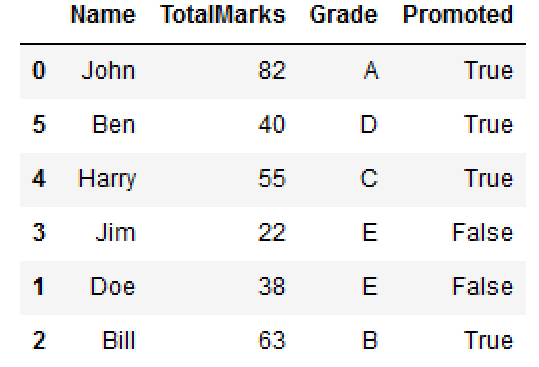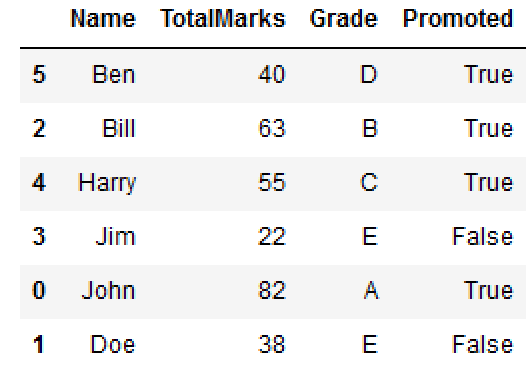DS Tutorial

# Randomly Shuffle DataFrame Rows in Pandas

In the scope of data manipulation using Pandas, a noteworthy capability that elevates the flexibility of data exploration and analysis lies in the process of randomly shuffling DataFrame rows. This powerful operation empowers data professionals to reorder the rows of a DataFrame in a non-deterministic fashion, introducing an element of randomness that enables statistical significance and fosters robustness in subsequent analyses. You can use the following methods to shuffle DataFrame rows:

1. Using pandas
pandas.DataFrame.sample()
1. Using numpy
numpy.random.permutation()
1. Using sklearn
sklearn.utils.shuffle()

The act of randomly shuffling DataFrame rows entails rearranging the order of data observations in a manner that bears no predetermined pattern, offering an unbiased representation of the dataset. This randomness is particularly advantageous when dealing with data that might have inherent ordering or when preparing data for training machine learning models that benefit from diverse and unpredictable sequences.

Lets create a DataFrame..

import pandas as pd import numpy as np df = pd.DataFrame() df['Name'] = ['John', 'Doe', 'Bill','Jim','Harry','Ben'] df['TotalMarks'] = [82, 38, 63,22,55,40] df['Grade'] = ['A', 'E', 'B','E','C','D'] df['Promoted'] = [True, False,True,False,True,True] df
Name TotalMarks Grade Promoted 0 John 82 A True 1 Doe 38 E False 2 Bill 63 B True 3 Jim 22 E False 4 Harry 55 C True 5 Ben 40 D True

## pandas.DataFrame.sample()

By employing the sample() method with the frac parameter set to 1 (indicating that all rows should be included) and replace parameter set to False (to ensure that no row is repeated), data professionals can confidently shuffle the DataFrame rows. This method guarantees an equitable distribution of rows, fostering equitable representation of the dataset while preserving its inherent characteristics.

df.sample(frac=1)Name TotalMarks Grade Promoted 3 Jim 22 E False 0 John 82 A True 5 Ben 40 D True 1 Doe 38 E False 2 Bill 63 B True 4 Harry 55 C True

Argument frac=1 means return all rows.

If you wish to shuffle your dataframe in-place and reset the index, you could do e.g.

df = df.sample(frac=1).reset_index(drop=True)

By using the sample() method with the frac parameter set to 1 (indicating that all rows should be included) and replace parameter set to False (to ensure that no row is repeated), data professionals can confidently shuffle the DataFrame rows. This method guarantees an equitable distribution of rows, fostering equitable representation of the dataset while preserving its inherent characteristics.

## numpy.random.permutation()

tmpDF =df.iloc[np.random.permutation(df.index)].reset_index(drop=True) tmpDF
Name TotalMarks Grade Promoted 0 Jim 22 E False 1 John 82 A True 2 Ben 40 D True 3 Doe 38 E False 4 Bill 63 B True 5 Harry 55 C True

Here, specifying drop=True prevents .reset_index from creating a column containing the old index entries.

The significance of random shuffling extends beyond exploratory data analysis, as it plays a key role in cross-validation procedures, enabling rigorous model assessment and selection. By employing random shuffling in the context of cross-validation, data professionals can obtain unbiased estimations of model performance, thereby gaining a comprehensive understanding of model effectiveness on diverse datasets.

## sklearn.utils.shuffle()

from sklearn.utils import shuffle shuffle(df)Name TotalMarks Grade Promoted 5 Ben 40 D True 4 Harry 55 C True 1 Doe 38 E False 3 Jim 22 E False 0 John 82 A True 2 Bill 63 B True

### Conclusion

The ability to randomly shuffle DataFrame rows in Pandas exemplifies the adaptability and versatility of this data manipulation library. The randomness introduced by this operation promotes data integrity, unbiased analyses, and robust model assessment, enriching the data analysis landscape with a layer of unpredictability that unveils new insights and strengthens data-driven decision-making processes. By using the power of random shuffling, data professionals can confidently navigate the complexities of their datasets and unravel meaningful patterns that inspire innovative solutions and drive transformative outcomes.